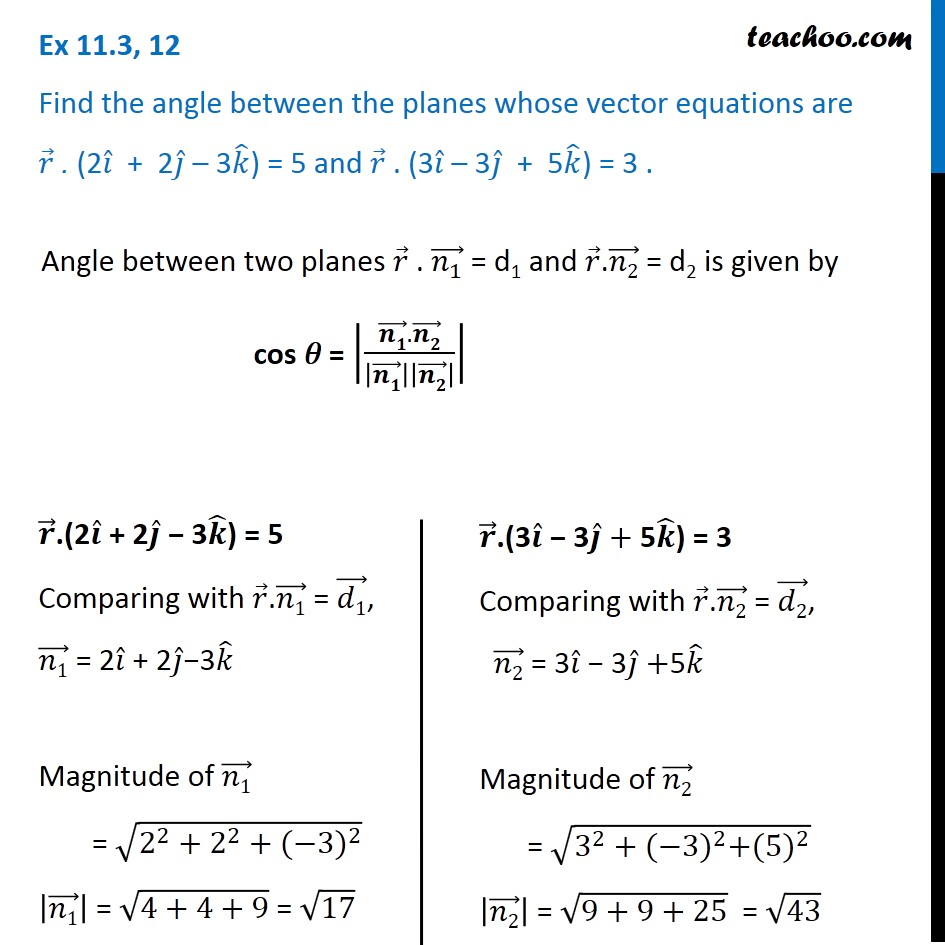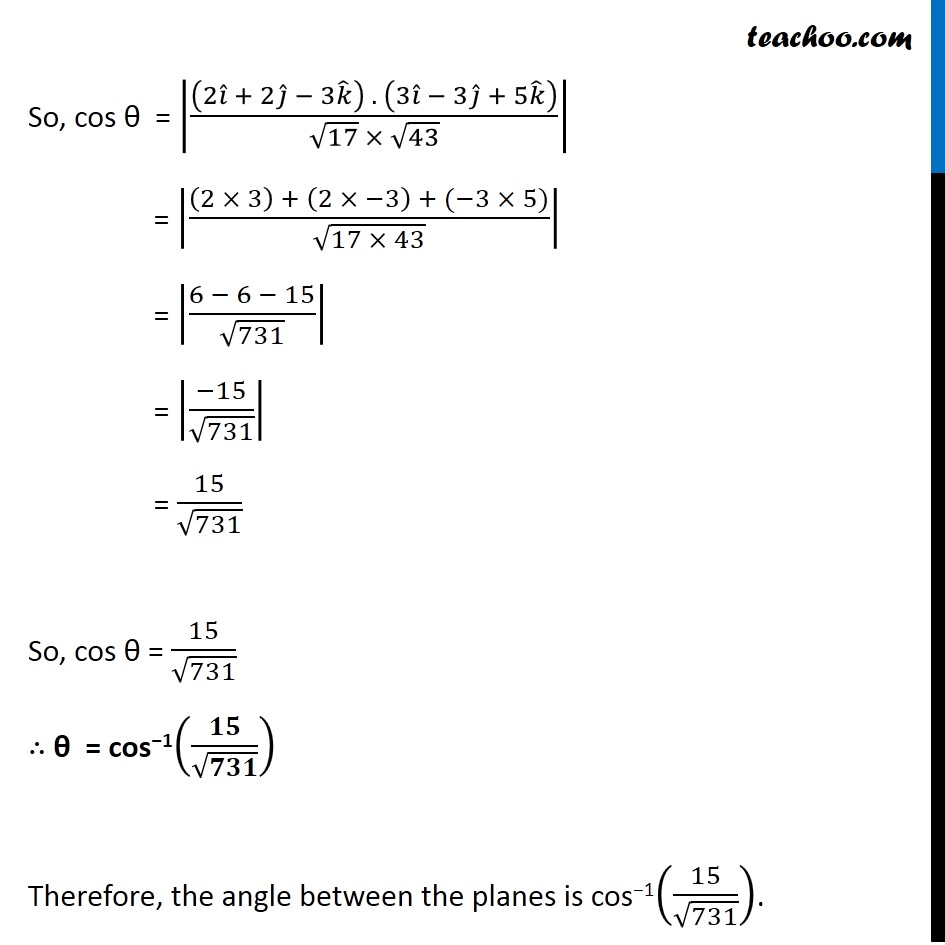Plane

Chapter 11 Class 12 Three Dimensional Geometry
Serial order wiseLearn in your speed, with individual attention - Teachoo Maths 1-on-1 Class

### Transcript

Question 12 Find the angle between the planes whose vector equations are 𝑟 ⃗ . (2𝑖 ̂ + 2𝑗 ̂ – 3𝑘 ̂) = 5 and 𝑟 ⃗ . (3𝑖 ̂ – 3𝑗 ̂ + 5𝑘 ̂) = 3 .Angle between two planes 𝑟 ⃗ . (𝑛_1 ) ⃗ = d1 and 𝑟 ⃗.(𝑛2) ⃗ = d2 is given by cos 𝜃 = |((𝒏𝟏) ⃗. (𝒏𝟐) ⃗)/|(𝒏𝟏) ⃗ ||(𝒏𝟐) ⃗ | | 𝒓 ⃗.(2𝒊 ̂ + 2𝒋 ̂ − 3𝒌 ̂) = 5 Comparing with 𝑟 ⃗.(𝑛1) ⃗ = (𝑑1) ⃗, (𝑛1) ⃗ = 2𝑖 ̂ + 2𝑗 ̂−3𝑘 ̂ Magnitude of (𝑛1) ⃗ = √(2^2+2^2+〖(−3)〗^2 ) |(𝑛1) ⃗ | = √(4+4+9) = √17 So, cos θ = |((2𝑖 ̂ + 2𝑗 ̂ − 3𝑘 ̂ ) . (3𝑖 ̂ − 3𝑗 ̂ + 5𝑘 ̂ ))/(√17 × √43)| = |((2 × 3) + (2 × −3) + (−3 × 5))/√(17 × 43)| = |(6 − 6 − 15)/√731| = |(−15)/√731| = 15/√731 So, cos θ = 15/√731 ∴ θ = cos−1(𝟏𝟓/√𝟕𝟑𝟏) Therefore, the angle between the planes is cos−1(15/√731).